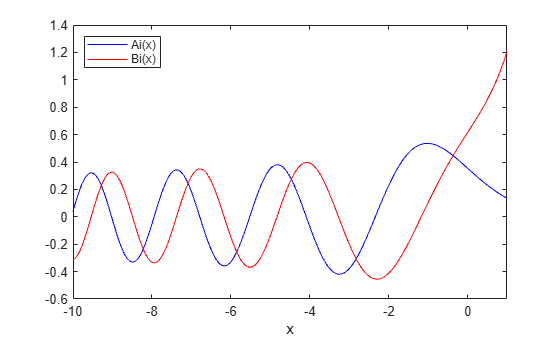# airy

Airy Functions

## Syntax

``W = airy(Z)``
``W = airy(k,Z)``
``W = airy(k,Z,scale)``

## Description

example

````W = airy(Z)` returns the Airy function, Ai(Z), for each element of Z.```

example

````W = airy(k,Z)` returns any of four different Airy functions, depending on the value of `k`, such as the Airy function of the second kind or the first derivative of an Airy function.```

example

````W = airy(k,Z,scale)` scales the resulting Airy function. `airy` applies a specific scaling function to `W` depending on your choice of `k` and `scale`.```

## Examples

collapse all

Define `x`.

`x = -10:0.01:1;`

Calculate Ai(x)

`ai = airy(x);`

Calculate Bi(x) using $k=2$.

`bi = airy(2,x);`

Plot both results together on the same axes.

```figure plot(x,ai,'-b',x,bi,'-r') axis([-10 1 -0.6 1.4]) xlabel('x') legend('Ai(x)','Bi(x)','Location','NorthWest')```Compute the Airy function at a slice through the complex plane at $x+i$.

Take a slice through the complex plane.

```x = -4:0.1:4; z = x+1i;```

Calculate Ai(z).

`w = airy(z);`

Plot the real part of the result.

```figure plot(x, real(w)) axis([-4 4 -1.5 1]) xlabel('real(z)')```Define `x`.

`x = -10:0.01:1;`

Calculate the scaled and unscaled Airy function.

```scaledAi = airy(0,x,1); noscaleAi = airy(0,x,0);```

Plot the real part of each result.

```rscaled = real(scaledAi); rnoscale = real(noscaleAi); figure plot(x,rscaled,'-b',x,rnoscale,'-r') axis([-10 1 -0.60 0.60]) xlabel('x') legend('scaled','not scaled','Location','SouthEast')```## Input Arguments

collapse all

System variable, specified as a real or complex vector, matrix, or N-D array.

Data Types: `single` | `double`
Complex Number Support: Yes

Type of Airy function, specified as one of four values.

k

Returns

`0`

Airy function,$Ai\left(Z\right)$, which is the same as `airy(Z)`.

`1`

First derivative of Airy function, $A{i}^{\prime }\left(Z\right)$.

`2`

Airy function of the second kind,$Bi\left(Z\right)$

`3`

First derivative of Airy function of the second kind,$B{i}^{\prime }\left(Z\right)$

Data Types: `single` | `double`

Scaling option, specified as `0` or `1`. Use `scale = 1` to enable the scaling of `Z`. The values you specify for `k` and `scale` determine the scaling function `airy` applies to `Z`.

scalekScaling applied to output
`0`Any

None

`1``0` or `1`

`${e}^{\frac{2}{3}{Z}^{\left(3/2\right)}}$`

`1``2` or `3`

`${e}^{-|\frac{2}{3}Re\left({Z}^{\left(3/2\right)}\right)|}$`

Data Types: `single` | `double`

## Output Arguments

collapse all

Airy function of `Z`, returned as an array the same size as `Z`.

collapse all

### Airy Functions

The Airy functions form a pair of linearly independent solutions to

`$\frac{{d}^{2}W}{d{Z}^{2}}-ZW=0.$`

The relationship between the Airy and modified Bessel functions is

`$\begin{array}{l}Ai\left(Z\right)=\left[\frac{1}{\pi }\sqrt{\frac{Z}{3}}\right]{K}_{1/3}\left(\zeta \right)\\ Bi\left(Z\right)=\sqrt{\frac{Z}{3}}\text{\hspace{0.17em}}\text{\hspace{0.17em}}\left[{I}_{-1/3}\left(\zeta \right)+{I}_{1/3}\left(\zeta \right)\right],\end{array}$`

where

`$\zeta =\frac{2}{3}{Z}^{3/2}.$`

## Version History

Introduced before R2006a